# Coulombs Law Coulombs law states that the force

• Slides: 26Coulomb’s Law Coulomb’s law states that the force of a charged object on another charged object is directly proportional to the product of their charge(s) and inversely proportional to the square of the distance between them.A student suspends a small piece of aluminum foil by a light insulating thread, holds the foil between her fingers for a moment, then releases it. The aluminum foil is then attracted towards a charged comb. Before it actually touches the comb, the foil _____. A. is neutral B. has the same charge as the comb C. has a charge opposite to the comb’sA small piece of aluminum foil is suspended by a light insulating thread. If you are explaining why the aluminum foil is attracted to a charged comb, which of the following should not be part of your explanation. A. Opposite charges attract, B. Like charges repel. C. The foil acquires an opposite charge from the comb. D. Charge can travel in conductors. E. Charges exert less force on each other when further apart.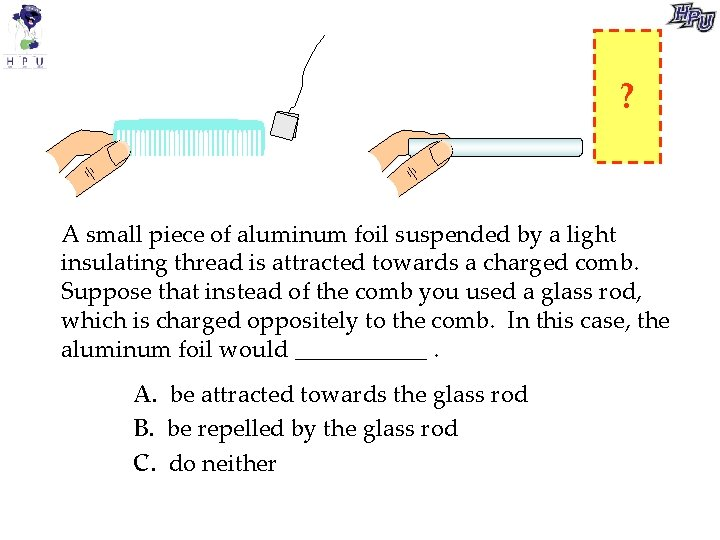? A small piece of aluminum foil suspended by a light insulating thread is attracted towards a charged comb. Suppose that instead of the comb you used a glass rod, which is charged oppositely to the comb. In this case, the aluminum foil would ______. A. be attracted towards the glass rod B. be repelled by the glass rod C. do neither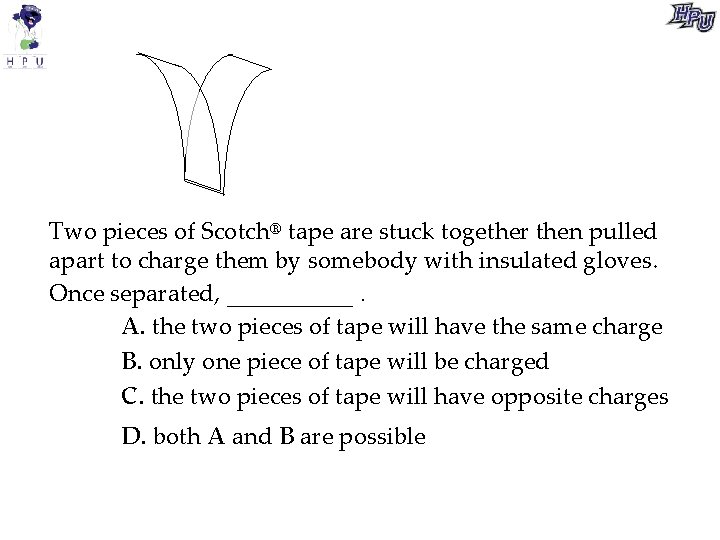Two pieces of Scotch® tape are stuck together then pulled apart to charge them by somebody with insulated gloves. Once separated, _____. A. the two pieces of tape will have the same charge B. only one piece of tape will be charged C. the two pieces of tape will have opposite charges D. both A and B are possible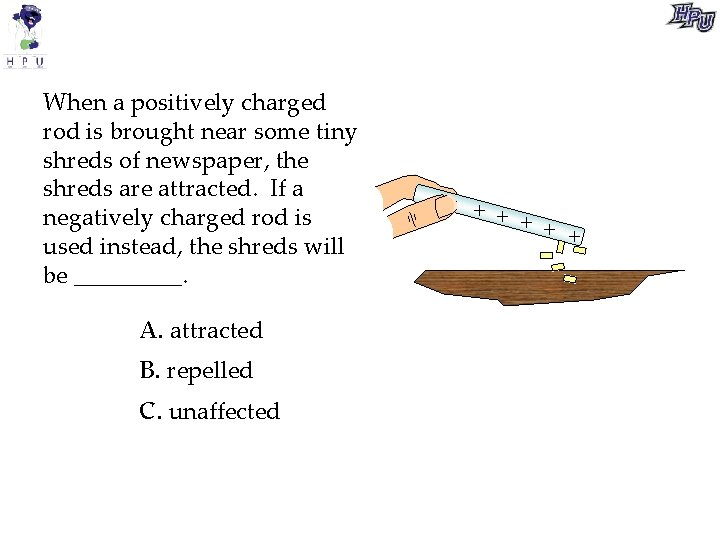When a positively charged rod is brought near some tiny shreds of newspaper, the shreds are attracted. If a negatively charged rod is used instead, the shreds will be _____. A. attracted B. repelled C. unaffected + + +B A A positively charged rod is brought toward two small conducting spheres suspended by insulating cords. Sphere A is attracted to the rod; sphere B is repelled by it. a. This shows that A initially _____ A. must have been positively charged. B. must have been neutral. C. must have been negatively charged. D. could have been positively charged or neutral. E. could have been negatively charged or neutral.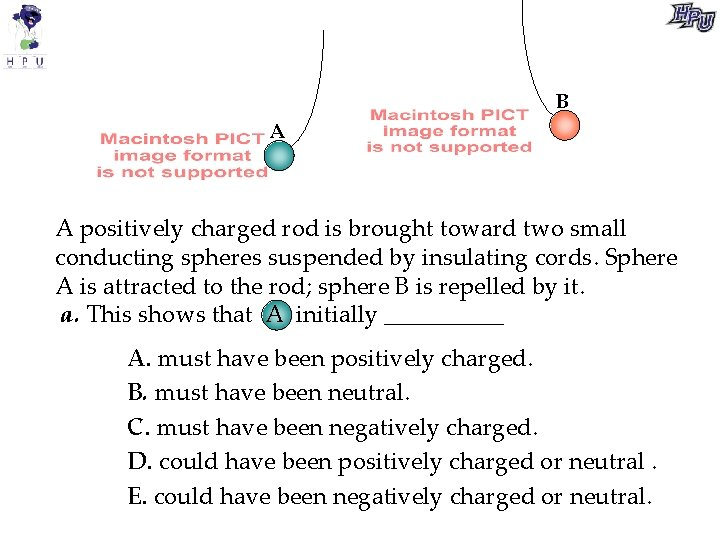B A A positively charged rod is brought toward two small conducting spheres suspended by insulating cords. Sphere A is attracted to the rod; sphere B is repelled by it. a. This shows that A initially _____ A. must have been positively charged. B. must have been neutral. C. must have been negatively charged. D. could have been positively charged or neutral. E. could have been negatively charged or neutral.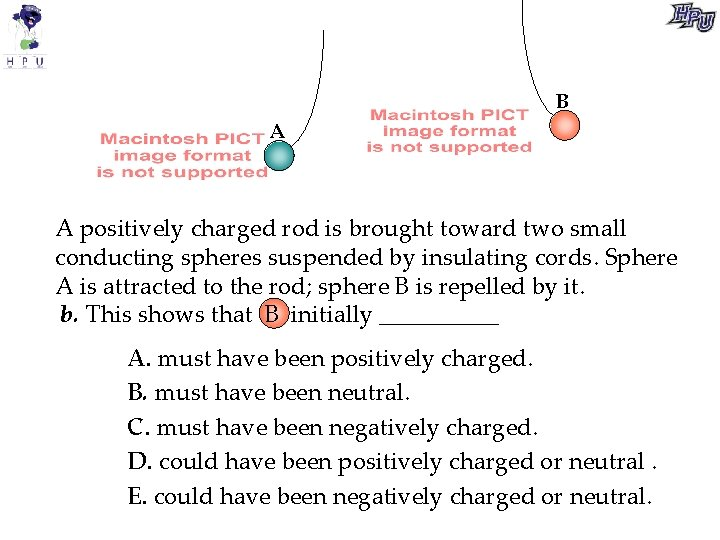B A A positively charged rod is brought toward two small conducting spheres suspended by insulating cords. Sphere A is attracted to the rod; sphere B is repelled by it. b. This shows that B initially _____ A. must have been positively charged. B. must have been neutral. C. must have been negatively charged. D. could have been positively charged or neutral. E. could have been negatively charged or neutral.Surface Charge In a conductor, excess charge will always be on the surface. This is called surface charge. The amount of surface charge per unit area is called surface charge density.Battery A battery acts like an “electron pump” that creates excess electrons on the - terminal and a deficit of electrons on the + terminal.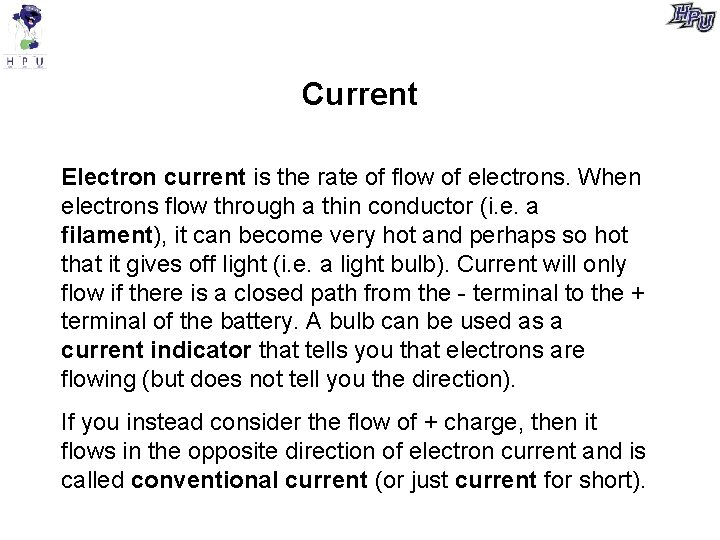Current Electron current is the rate of flow of electrons. When electrons flow through a thin conductor (i. e. a filament), it can become very hot and perhaps so hot that it gives off light (i. e. a light bulb). Current will only flow if there is a closed path from the - terminal to the + terminal of the battery. A bulb can be used as a current indicator that tells you that electrons are flowing (but does not tell you the direction). If you instead consider the flow of + charge, then it flows in the opposite direction of electron current and is called conventional current (or just current for short).Direction of electron current in a simple circuit of a battery and bulbDirection of conventional current in a simple circuit of a battery and bulb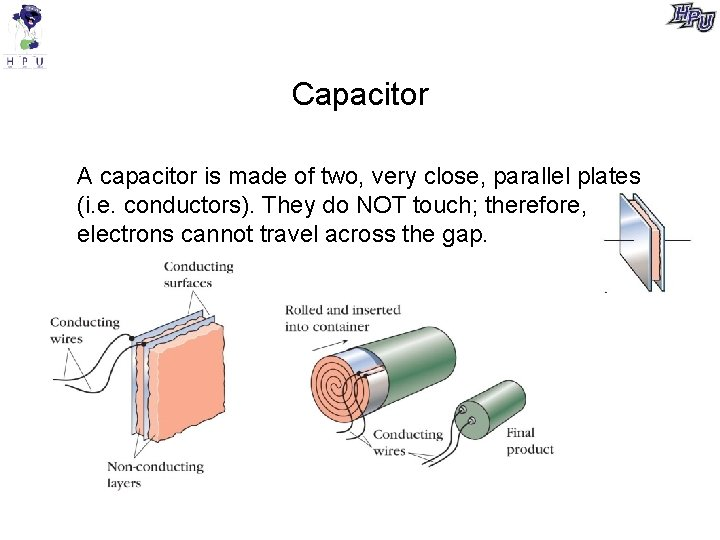Capacitor A capacitor is made of two, very close, parallel plates (i. e. conductors). They do NOT touch; therefore, electrons cannot travel across the gap.Battery, bulb, and capacitor At t=0, the clips are connected. Describe the electron current at t=0 and the electron current after a long time.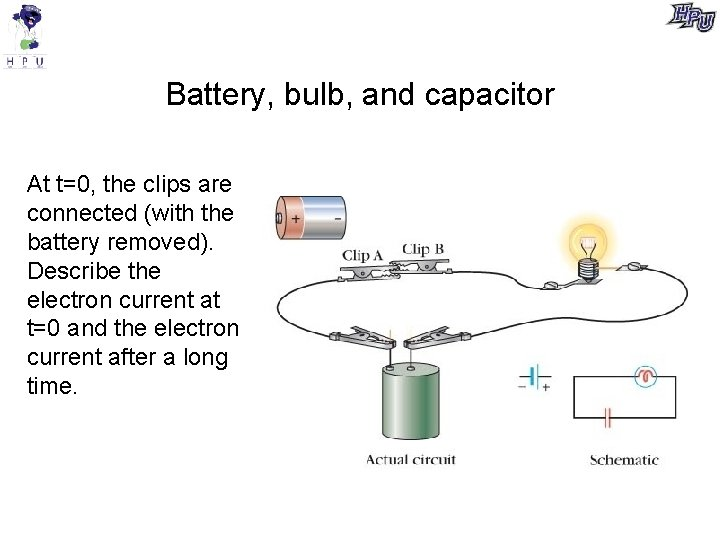Battery, bulb, and capacitor At t=0, the clips are connected (with the battery removed). Describe the electron current at t=0 and the electron current after a long time.When the bulb shown here is connected just to the battery, it glows. In this circuit, though, a capacitor is included in series with the bulb and battery. When the last connection is made, the bulb will ___________. A. glow with gradually increasing brightness B. glow with the same constant brightness as without the capacitor C. glow with constant brightness but more dimly than without the capacitor D. glow with gradually decreasing brightness E. not glow at all.battery bulb capacitor As a capacitor is charged in a circuit like this, ________. A. electrons leave the negative plate at a constant rate B. electrons leave the positive plate at a constant rate C. it becomes increasingly difficult for electrons to leave the negative plate D. it becomes increasingly difficult for electrons to leave the positive plate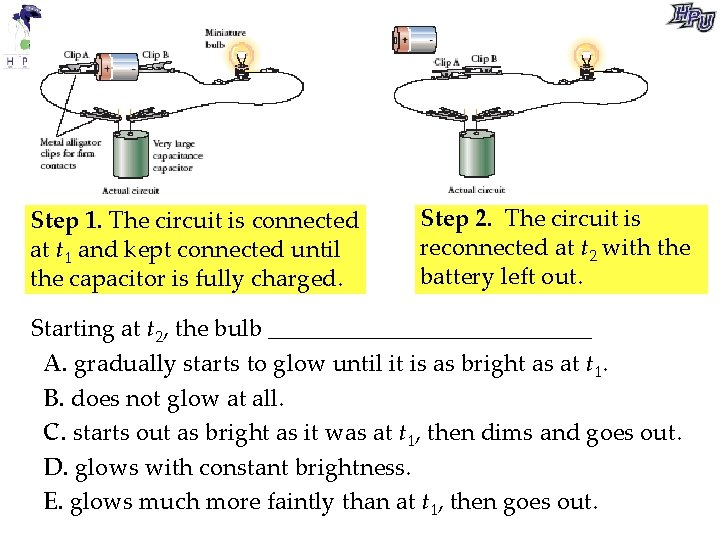Step 1. The circuit is connected at t 1 and kept connected until the capacitor is fully charged. Step 2. The circuit is reconnected at t 2 with the battery left out. Starting at t 2, the bulb ______________ A. gradually starts to glow until it is as bright as at t 1. B. does not glow at all. C. starts out as bright as it was at t 1, then dims and goes out. D. glows with constant brightness. E. glows much more faintly than at t 1, then goes out.How does the bulb affect how long it takes to charge the capacitor? Change the bulb to a different type. Why does it affect how long it takes to charge the capacitor? The total charge on the capacitor plates after a “long time” (called maximum charge) is the same no matter which bulb is being used (after all, the filament is just a conductor that allows electrons to flow through it). So, the bulb affects the rate that electrons flow. Though one bulb, electrons flow fast (i. e. high current). Through the other bulb, electrons flow slow (i. e. low current). This quality of the bulb is called resistance.You are about to charge a capacitor using the circuit shown. Two bulbs are available for use in the circuit. Bulb A has higher resistance and bulb B has lower resistance. Charging the capacitor through bulb A will use ________ charging the capacitor through bulb B. A. more total energy than B. the same total energy as C. less total energy than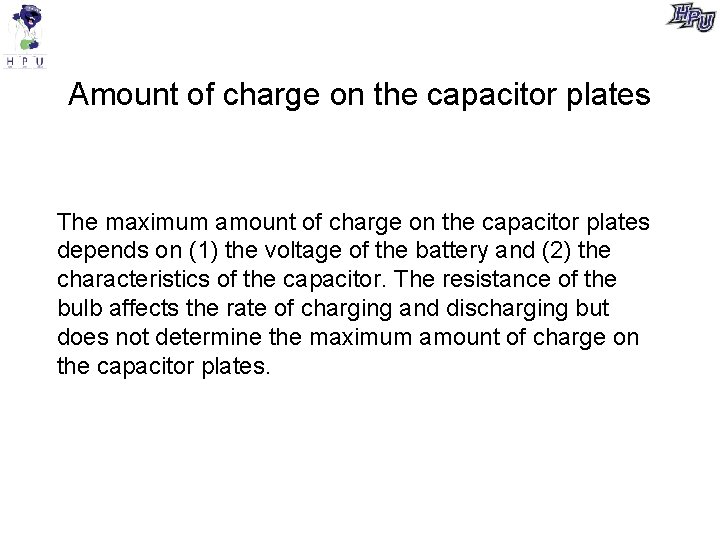Amount of charge on the capacitor plates The maximum amount of charge on the capacitor plates depends on (1) the voltage of the battery and (2) the characteristics of the capacitor. The resistance of the bulb affects the rate of charging and discharging but does not determine the maximum amount of charge on the capacitor plates.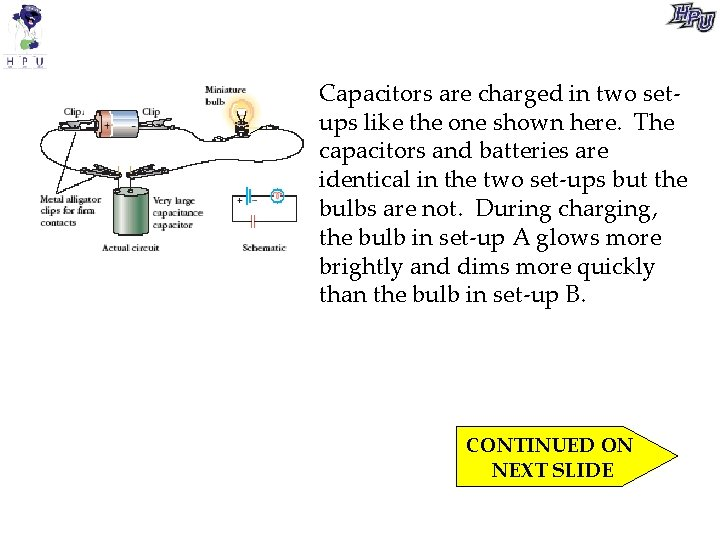Capacitors are charged in two setups like the one shown here. The capacitors and batteries are identical in the two set-ups but the bulbs are not. During charging, the bulb in set-up A glows more brightly and dims more quickly than the bulb in set-up B. CONTINUED ON NEXT SLIDE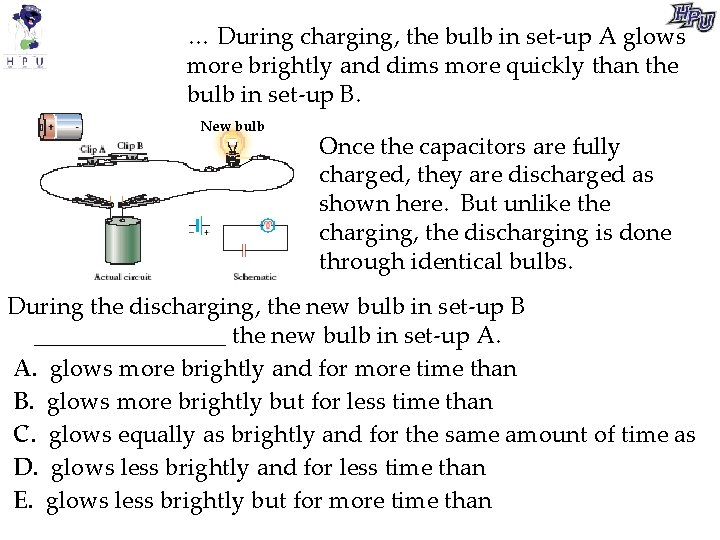… During charging, the bulb in set-up A glows more brightly and dims more quickly than the bulb in set-up B. New bulb Once the capacitors are fully charged, they are discharged as shown here. But unlike the charging, the discharging is done through identical bulbs. During the discharging, the new bulb in set-up B ________ the new bulb in set-up A. A. glows more brightly and for more time than B. glows more brightly but for less time than C. glows equally as brightly and for the same amount of time as D. glows less brightly and for less time than E. glows less brightly but for more time than